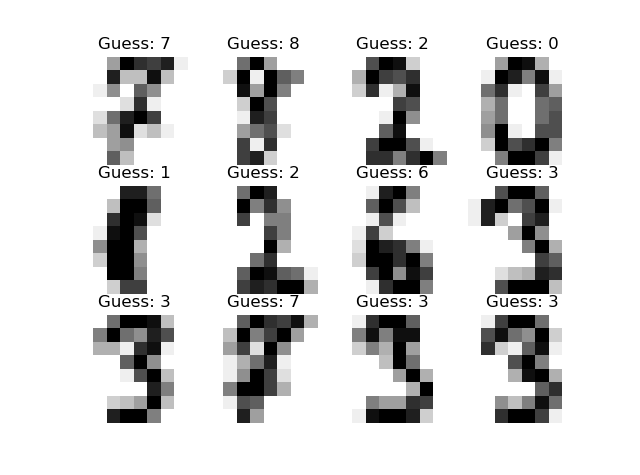# Introduction

① https://qiita.com/yohiro/items/04984927d0b455700cd1 ② https://qiita.com/yohiro/items/5aab5d28aef57ccbb19c ③ https://qiita.com/yohiro/items/cc9bc2631c0306f813b5 ④ https://qiita.com/yohiro/items/d376f44fe66831599d0b ⑤ https://qiita.com/yohiro/items/3abaf7b610fbcaa01b9c Continued

--Reference materials: Udemy Everyone's AI course Artificial intelligence and machine learning learned from scratch with Python --Library used: scikit-learn

# Issue setting

Recognize the written numbers from the handwritten number image (8 x 8 px).

# Source code

## import

``````from sklearn import datasets
from sklearn import svm
from sklearn import metrics
import matplotlib.pyplot as plt
``````

``````#Reading numeric data
``````

The digits contains the following data.

#### `digits.data`

``````
[[ 0.  0.  5. ...  0.  0.  0.]
[ 0.  0.  0. ... 10.  0.  0.]
[ 0.  0.  0. ... 16.  9.  0.]
...
[ 0.  0.  1. ...  6.  0.  0.]
[ 0.  0.  2. ... 12.  0.  0.]
[ 0.  0. 10. ... 12.  1.  0.]]
``````

#### `digits.target`

``````
[0 1 2 ... 8 9 8]
``````

`digits.data` is a 64x1797 list, the element values represent colors in grayscale, and one 64 element list represents one image. For image display, `digits.image` also contains the same information although the list format is different. `digits.target` shows the correct answer (= which number is represented) of each image.

## Training with support vector machine

``````#Support vector machine
clf = svm.SVC(gamma=0.001, C=100.0) # gamma:The magnitude of the impact of one training data, C:False recognition tolerance
#Training with support vector machine(60% of data is used, the remaining 40% is for verification)
clf.fit(digits.data[:int(n*6/10)], digits.target[:int(n*6/10)])
``````

The last time I used it was `LinearSVC ()`, but this time I'm using `SVC ()`. Is it not possible to classify by linear boundaries?

## Classification

Have the clf created above read the remaining 40% of the data in digits.data and classify each number.

``````#Correct answer
expected = digits.target[int(-n*4/10):]
#Forecast
predicted = clf.predict(digits.data[int(-n*4/10):])
print(metrics.classification_report(expected, predicted))
#Misrecognition matrix
print(metrics.confusion_matrix(expected, predicted))
``````

## result

``````              precision    recall  f1-score   support

0       0.99      0.99      0.99        70
1       0.99      0.96      0.97        73
2       0.99      0.97      0.98        71
3       0.97      0.86      0.91        74
4       0.99      0.96      0.97        74
5       0.95      0.99      0.97        71
6       0.99      0.99      0.99        74
7       0.96      1.00      0.98        72
8       0.92      1.00      0.96        68
9       0.96      0.97      0.97        71

accuracy                           0.97       718
macro avg       0.97      0.97      0.97       718
weighted avg       0.97      0.97      0.97       718
``````

99% of the answers predicted to be 0 are correct, and 99% of the correct answers were predicted to be 0. Reference of how to read the table: -How to read classification_report -Be careful when putting F1 score in metarcs with Keras

### Misrecognition matrix

``````[[69  0  0  0  1  0  0  0  0  0]
[ 0 70  1  0  0  0  0  0  2  0]
[ 1  0 69  1  0  0  0  0  0  0]
[ 0  0  0 64  0  3  0  3  4  0]
[ 0  0  0  0 71  0  0  0  0  3]
[ 0  0  0  0  0 70  1  0  0  0]
[ 0  1  0  0  0  0 73  0  0  0]
[ 0  0  0  0  0  0  0 72  0  0]
[ 0  0  0  0  0  0  0  0 68  0]
[ 0  0  0  1  0  1  0  0  0 69]]
``````

Of the 0 images, 69 are recognized as 0, 1 is recognized as 4, and so on.

## Actual image and predicted value

``````#Correspondence between prediction and image (part)
images = digits.images[int(-n*4/10):]
for i in range(12):
plt.subplot(3, 4, i + 1)
plt.axis("off")
plt.imshow(images[i], cmap=plt.cm.gray_r, interpolation="nearest")
plt.title("Guess: " + str(predicted[i]))
plt.show()
``````You can see that the numbers can be recognized.

# bonus

I tried to visualize digits.data (black and white binary image)

``````for i in range(10):
my_s = ""
for k, j in enumerate(digits.data[i]):
if (j > 0):
my_s += " ■ "
else:
my_s += "   "
if k % 8 == 7:
print(my_s)
my_s = ""
print("\n")
``````

result

``````       ■  ■  ■  ■
■  ■  ■  ■  ■
■  ■  ■     ■  ■
■  ■        ■  ■
■  ■        ■  ■
■  ■     ■  ■  ■
■  ■  ■  ■  ■
■  ■  ■

■  ■  ■
■  ■  ■
■  ■  ■  ■
■  ■  ■  ■  ■
■  ■  ■  ■
■  ■  ■  ■
■  ■  ■  ■
■  ■  ■

...

■  ■  ■  ■
■  ■  ■  ■
■  ■     ■  ■
■  ■  ■  ■  ■
■  ■  ■  ■
■  ■  ■  ■  ■  ■
■  ■  ■  ■  ■  ■
■  ■  ■  ■  ■

■  ■
■  ■  ■  ■  ■
■  ■  ■  ■  ■
■  ■  ■  ■  ■
■  ■  ■  ■  ■
■     ■  ■
■  ■  ■
■  ■  ■  ■
``````

You can see that it is handwritten somehow